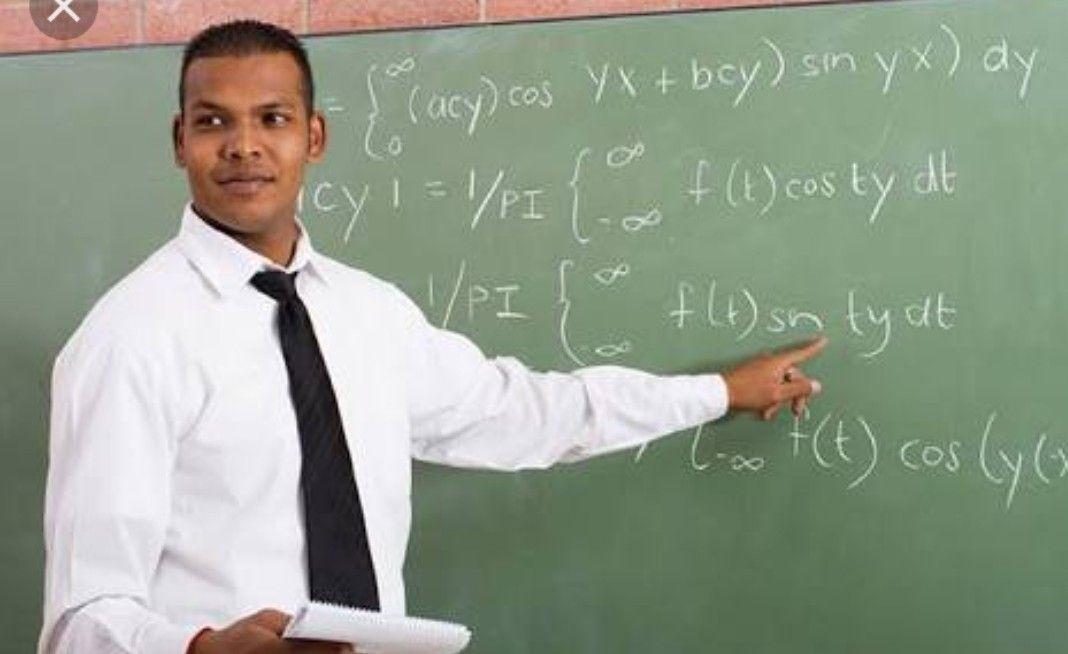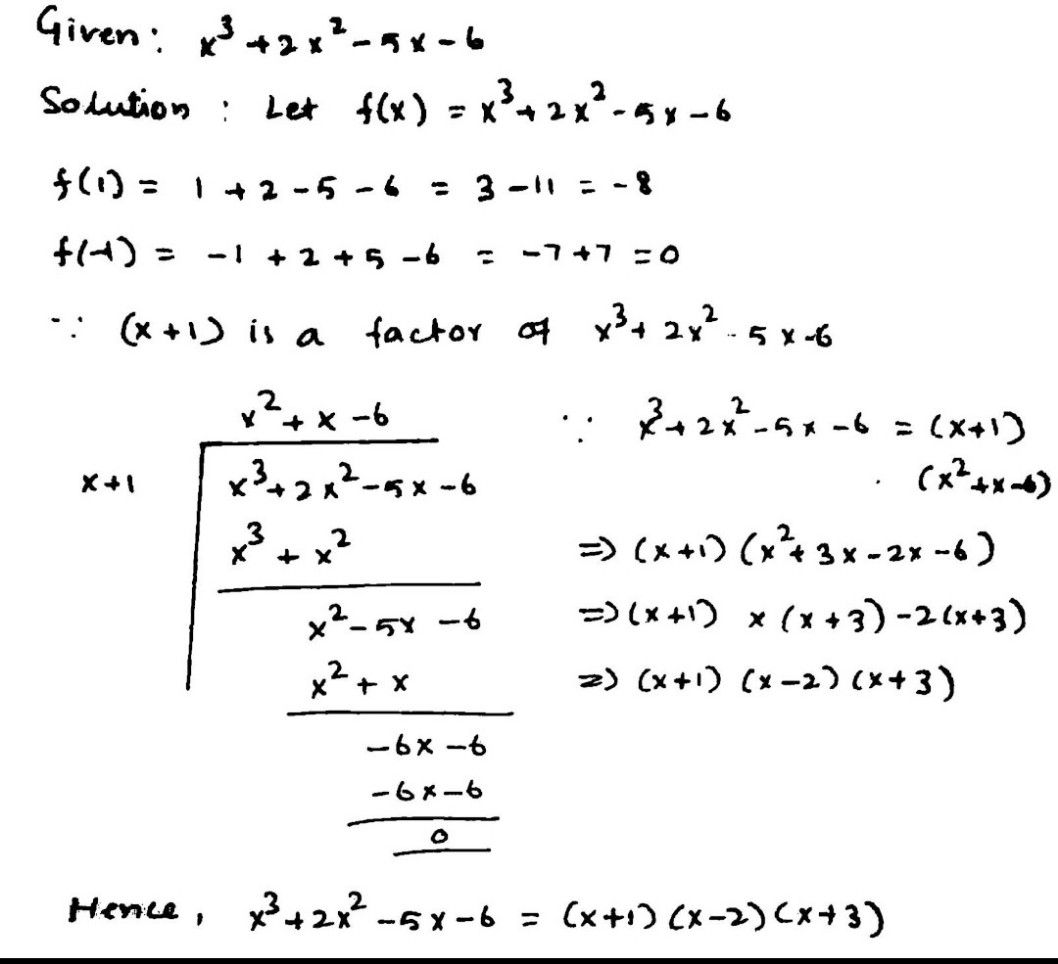Symbol
Problem$2$ Learning Tasak $2$ A. Determine the $\left(2cta=$ of the given polynomial. $1.x3+2x2-5x-6$ $2$ $x3.x2-x-1$ $3$ $x3-x2-10x-8$
10th-13th grade
Other
SolutionQanda teacher - SUDESH